# How to use the Excel IF Function

The IF function in Excel is used to give conditional outputs.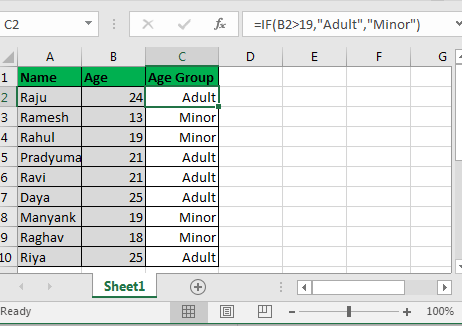Syntax:

 =IF(condition, value if TRUE, value if FALSE)

The IF statement in Excel checks the condition and returns the specified value if the condition is TRUE and another specified value if FALSE. At the place of value if TRUE and value of FALSE, you can put a value, a text within quotes, another formula or even another if statement (nested IF statement, we will talk about it).

 Pro Note: IF in Excel 2016, 2013, and 2010 can have up to 64 nested IF statements. In Excel 2007 it was only 7.

The best thing about the IF statement is that you can customise TRUE and FALSE results. And this is what it is used for. Let’s see how...

# IF Example 1:

Assume you have a list of people. Now you want to know how many of them are adults and how many are minors.

Let's say people whose age is greater than 19 are Adults and who are less than 19 are minor.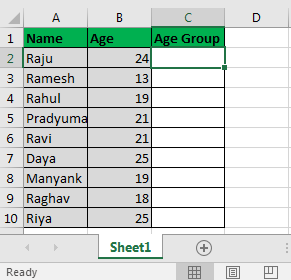In Cell C2 write this excel IF statement and drag it down:

Here, Excel will simply check if the value in cell B2 is greater than 19 or not. Since it is greater than 19, it shows Adult in C2. And it does the same for all cells. Finally, we get this:This was a simple example of an IF function in Excel. However, most of the time you would require nested IF or a combination of IF with other Excel functions.

Let's have another example of the IF statement.

# Example 2. Nested IF in Excel To Check Multiple Conditions

Assume, in a given list, you are required to tell if a kid is an Adult or “Teenager or KID”. And if Minor is then he is a teenager (between the age of 13 and 19) or a kid (below the age of 13).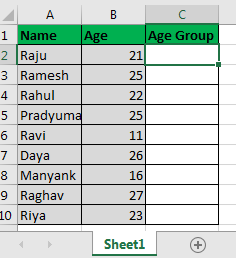So here we have to do this

IF (is student’s age <20, if yes checkif(is student’s age <13, if yes then show “Kid”, If no then show “Teenager”), if No then show “Adult”)

There are other ways to do it but for the sake of understanding, we are taking this example.

```Info: Most formulas are solved inside out but not IF statements.
In a nested IF function, outer IF is solved first and then the inner IF.
this is a basic diagram of the nested IFs control flow.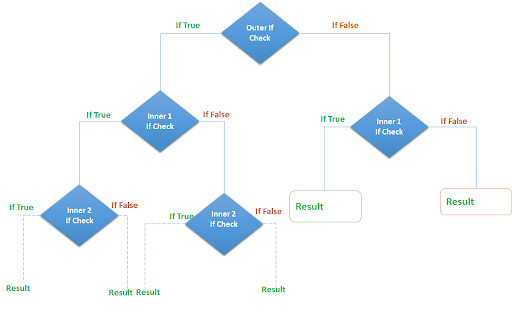```

In cell C2, write this IF formula and drag it down to cell C10:

This is the final product we will have.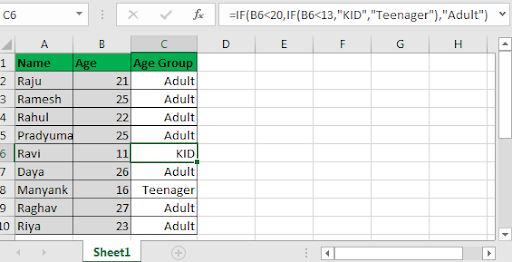Now, let's understand this. It's easy.

IF(B6<20: this statement checks if the value in B6 is less than 20 not.

Since it is not, it skips the Value IF TRUE (IF(B6<13,"KID","Teenager"))  part and jumps to Value IF FALSE part and shows “Adult”.

Since most of them are above or equal to the age of 20, they are shown as “Adult”.

Note that Ravi is shown as “KID” as his age is 11 and Manyank is shown as “Teenager” as his age 16.

First, excel checks if Ravi’s age is <20. It’s TRUE. The control then moves to a TRUE section that contains another IF statement IF(B6<13. Next

Excel checks if Ravi<13. It’s TRUE. Control moves to the TRUE section of the IF. It contains “KID” and therefore it shows “KID” there.

Important Notes:

1. Nested IFs are solved inwards. The outer IF acts as a gateway to inner IF.
2. In Excel 2016, 2013 and 2010, you can have up to 64 steps of IF statements. In earlier Excel versions, it was only 7.
3. IF supports logical these operators = (equals to),< (less than), > (greater than) ,<= (less than or equal to),>= (greater than or equal to, <> (not equal to)
4. FALSE statements are optional, but TRUE options are mandatory.

Related Articles:

IF function with Wildcards

IF with OR Function in Excel

IF with AND Function in Excel

Popular Articles:

50 Excel Shortcut to Increase Your Productivity

How to use the VLOOKUP Function in Excel

How to use the COUNTIF function in Excel 2016

How to Use SUMIF Function in Excel

Terms and Conditions of use

The applications/code on this site are distributed as is and without warranties or liability. In no event shall the owner of the copyrights, or the authors of the applications/code be liable for any loss of profit, any problems or any damage resulting from the use or evaluation of the applications/code.